Zen Pharaohs of Ultimate Love mathematics, music, science, wood....what else could you ask?Next: Maximally Flat Impulse Response Up: Recursive Implementation of General Previous: Recursive Implementation of General

## Interpolation and Irregularly Spaced Data

We can generalize this recursive realization to the case where the times at which data arrives are not equally spaced points. In this case we put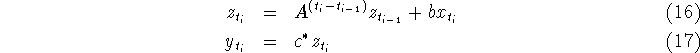with impulse response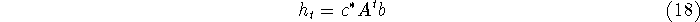as before. Note that if the times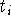are successive integers, that is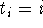, then this filter coincides with (15). We note that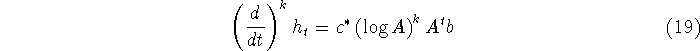permits us to compute derivatives of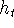, where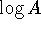is the matrix logarithm. The DC gain for irregularly spaced data depends on the sequence of times, so that a gain-normalized filter requires a method of keeping track of these times. One convenient approach is to approximate the normalized filter by adjoining extra statesFri Jun 27 03:10:38 EDT 1997